×#### Thank you for registering.

One of our academic counsellors will contact you within 1 working day.

Click to Chat

1800-1023-196

+91-120-4616500

CART 0

• 0

MY CART (5)

Use Coupon: CART20 and get 20% off on all online Study Material

ITEM
DETAILS
MRP
DISCOUNT
FINAL PRICE
Total Price: Rs.

There are no items in this cart.
Continue Shopping• Complete JEE Main/Advanced Course and Test Series
• OFFERED PRICE: Rs. 15,900
• View Details

```Chapter 3: Functions – Exercise 3.4

Functions – Exercise 3.4 – Q.1

We have,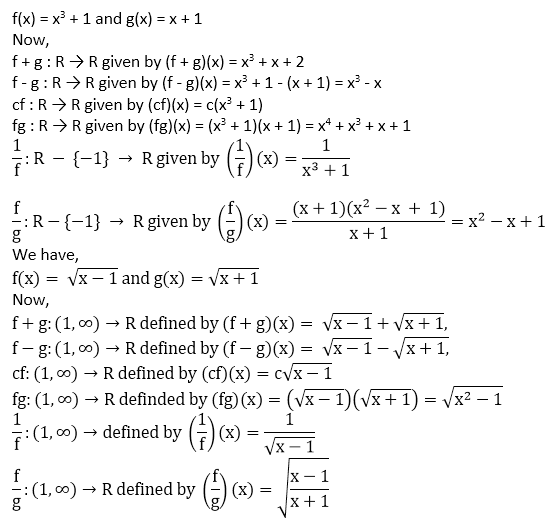Functions – Exercise 3.4 – Q.2

We have,

f(x) = 2x + 5 and g(x) = x2 + x

We observe that f(x) = 2x + 5 is defined for all x ϵ R

So, domain (g) = R

Clearly g(x) = x2 + x is defined for all x ϵ R

So, domain (g) = R

∴ Domain (f) ∩ Domain (g) = R

(i) Clearly, (f + g): R → R is given by

(f + g)(x) = f(x) + g(x)

= 2x + 5 + x2 + x

= x2 + 3x + 5

Domain (f + g) = R

(ii) We find that f - g : R → R is defined as

(f - g)(x) = f(x) - g(x)

= 2x + 5 - (x2 + x)

= 2x + 5 - x2 - x

= -x2 + x + 5

Domain (f - g) = R

(iii) We find that fg : R → R is given by

(fg)(x) = f(x) × g(x)

= (2x + 5) × (x2 + x)

= 2x3 + 2x2 + 5x2 + 5x

= 2x3 + 7x2 + 5x

Domain (fg) = R

(iv) we have,

g(x) = x2 + x

∴ f(x) = 0 ⟹ x2 + x = 0

⟹ x(x + 1) = 0

⟹ x = 0 or, x = -1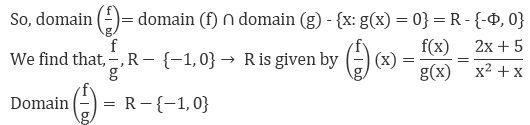Functions – Exercise 3.4 – Q.3

We have,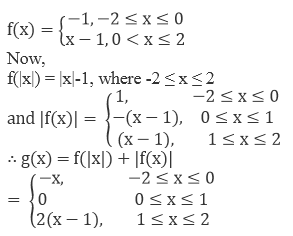Functions – Exercise 3.4 – Q.4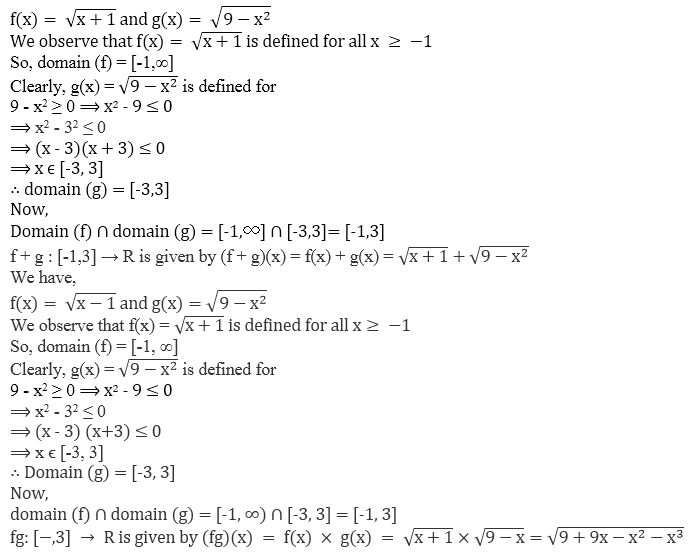We have,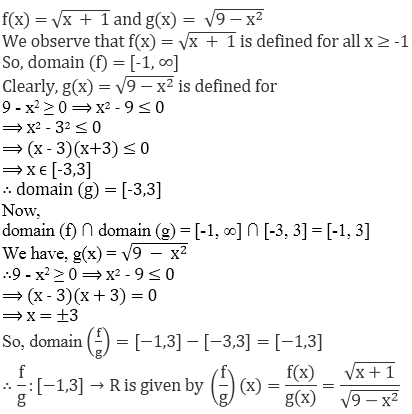We have,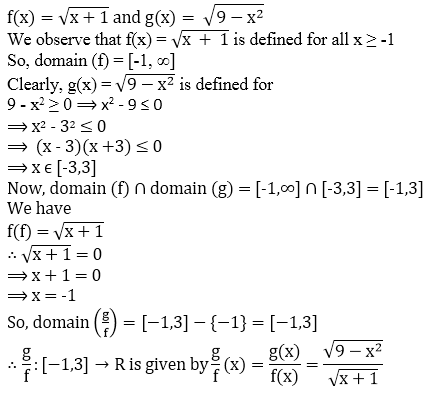We have,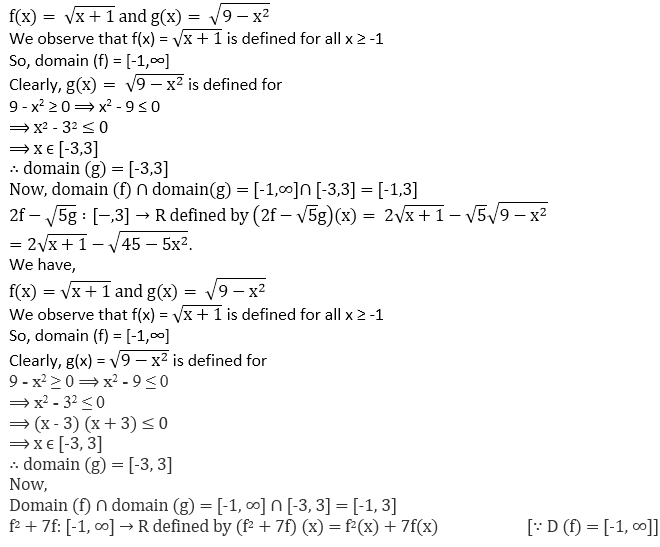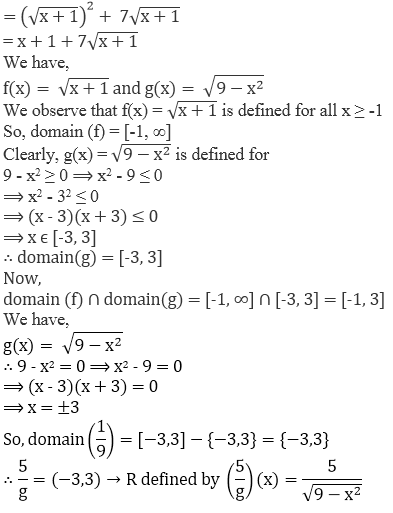```### Course Features

• 728 Video Lectures
• Revision Notes
• Previous Year Papers
• Mind Map
• Study Planner
• NCERT Solutions
• Discussion Forum
• Test paper with Video Solution